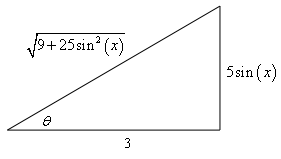Paul's Online Notes
Home / Calculus II / Integration Techniques / Trig Substitutions
Show Mobile Notice Show All Notes Hide All Notes
Mobile Notice
You appear to be on a device with a "narrow" screen width (i.e. you are probably on a mobile phone). Due to the nature of the mathematics on this site it is best views in landscape mode. If your device is not in landscape mode many of the equations will run off the side of your device (should be able to scroll to see them) and some of the menu items will be cut off due to the narrow screen width.

### Section 7.3 : Trig Substitutions

16. Use a trig substitution to evaluate $$\displaystyle \int{{\cos \left( x \right)\sqrt {9 + 25{{\sin }^2}\left( x \right)} \,dx}}$$.

Show All Steps Hide All Steps

Start Solution

Let’s first rewrite the integral a little bit.

$\int{{\cos \left( x \right)\sqrt {9 + 25{{\sin }^2}\left( x \right)} \,dx}} = \int{{\cos \left( x \right)\sqrt {9 + 25{{\left[ {\sin \left( x \right)} \right]}^2}} \,dx}}$ Show Step 2

With the integral written as it is in the first step we can now see that we do have a sum of a number and something squared under the root. We know from the problems done previously in this section that looks like a tangent substitution. So, let’s use the following substitution.

$\sin \left( x \right) = \frac{3}{5}\tan \left( \theta \right)$

Do not get excited about the fact that we are substituting one trig function for another. That will happen on occasion with these kinds of problems. Note however, that we need to be careful and make sure that we also change the variable from $$x$$ (i.e. the variable in the original trig function) into $$\theta$$ (i.e. the variable in the new trig function).

Next let’s eliminate the root.

$\sqrt {9 + 25{{\left[ {\sin \left( x \right)} \right]}^2}} = \sqrt {9 + 25{{\left[ {\frac{3}{5}\tan \left( \theta \right)} \right]}^2}} = \sqrt {9 + 9{{\tan }^2}\left( \theta \right)} = 3\sqrt {{{\sec }^2}\left( \theta \right)} = 3\left| {\sec \left( \theta \right)} \right|$

Next, because we are doing an indefinite integral we will assume that the secant is positive and so we can drop the absolute value bars to get,

$\sqrt {9 + 25{{\left[ {\sin \left( x \right)} \right]}^2}} = 3\sec \left( \theta \right)$

For a final substitution preparation step let’s also compute the differential so we don’t forget to use that in the substitution!

$\cos \left( x \right)\,dx = \frac{3}{5}{\sec ^2}\left( \theta \right)\,d\theta$

Recall that all we really need to do here is compute the differential for both the right and left sides of the substitution.

Show Step 3

Now let’s do the actual substitution.

\begin{align*}\int{{\cos \left( x \right)\sqrt {9 + 25{{\sin }^2}\left( x \right)} \,dx}} & = \int{{\sqrt {9 + 25{{\left[ {\sin \left( x \right)} \right]}^2}} \,\,\,\,\cos \left( x \right)dx}}\\ & = \int{{\left( {3\sec \left( \theta \right)} \right)\,\,\left( {\frac{3}{5}{{\sec }^2}\left( \theta \right)} \right)d\theta }} = \frac{9}{5}\int{{{{\sec }^3}\left( \theta \right)d\theta }}\end{align*}

Do not forget to substitute in the differential we computed in the previous step. This is probably the most common mistake with trig substitutions. Forgetting the differential can substantially change the problem, often making the integral very difficult to evaluate.

Show Step 4

We now need to evaluate the integral. Here is that work.

$\int{{\cos \left( x \right)\sqrt {9 + 25{{\sin }^2}\left( x \right)} \,dx}} = \frac{9}{{10}}\left[ {\sec \left( \theta \right)\tan \left( \theta \right) + \ln \left| {\sec \left( \theta \right) + \tan \left( \theta \right)} \right|} \right] + c$

Note that this was one of the few trig substitution integrals that didn’t really require a lot of manipulation of trig functions to completely evaluate. All we had to really do here was use the fact that we determined the integral of $${\sec ^3}\left( \theta \right)$$ in the previous section and reuse that result here.

Show Step 5

As the final step we just need to go back to $$x$$’s. To do this we’ll need a quick right triangle. Here is that work.

 From the substitution we have, $\tan \left( \theta \right) = \frac{{5\sin \left( x \right)}}{3}\,\,\,\,\,\left( { = \frac{{{\mbox{opp}}}}{{{\mbox{adj}}}}} \right)$ From the right triangle we get, $\sec \left( \theta \right) = \frac{{\sqrt {9 + 25{{\sin }^2}\left( x \right)} }}{3}$The integral is then,

$\int{{\cos \left( x \right)\sqrt {9 + 25{{\sin }^2}\left( x \right)} \,dx}} = \require{bbox} \bbox[2pt,border:1px solid black]{{\frac{{\sin \left( x \right)\sqrt {9 + 25{{\sin }^2}\left( x \right)} }}{2} + \frac{9}{{10}}\ln \left| {\frac{{5\sin \left( x \right) + \sqrt {9 + 25{{\sin }^2}\left( x \right)} }}{3}} \right| + c}}$